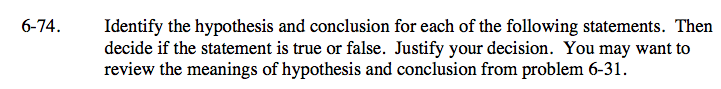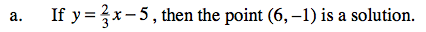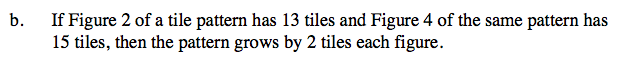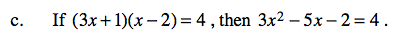### Home > CAAC > Chapter 6 > Lesson 6.2.4 > Problem6-74

6-74.Hypothesis:

$y=\frac{2}{3}x \minus 5$

Conclusion: (6, −1) is a solution

Check to see if the conclusion is true by plugging in the points into the original equation.Add 2 tiles to Figure 2 to find figure three. Repeat the process to find Figure 4.
Does the number of tiles you found for Figure 4 match the number that the problem says there should be?Hypothesis: (3x + 1)(x − 2) = 4
Conclusion: 3x2 − 5x − 2 = 4

Check by making a generic rectangle.
Does (3x + 1)(x − 2) = 3x2 − 5x − 2?